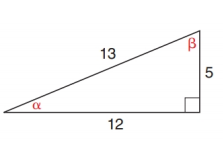Chapter 11.2, Problem 1E### Elementary Geometry for College St...

6th Edition
Daniel C. Alexander + 1 other
ISBN: 9781285195698

#### Solutions

Chapter
Section### Elementary Geometry for College St...

6th Edition
Daniel C. Alexander + 1 other
ISBN: 9781285195698
Textbook Problem
1 views

# In Exercises 1 to 6, find cos α and cos β .To determine

To find:

cosα and cosβ.

Explanation

Consider the following figure,

General formula for cosine ratio is given below,

From the given figure 13 is the hypotenuse value since it is opposite to right angle.

Then 12 is the length of the leg adjacent to α and 5 is the length of leg adjacent to β

### Still sussing out bartleby?

Check out a sample textbook solution.

See a sample solution

#### The Solution to Your Study Problems

Bartleby provides explanations to thousands of textbook problems written by our experts, many with advanced degrees!

Get Started

#### Sketch the graphs of the equations in Exercises 512. yx=1

Finite Mathematics and Applied Calculus (MindTap Course List)

#### Evaluate the integral. 20. tan5sec3d

Single Variable Calculus: Early Transcendentals

#### True or false:

Study Guide for Stewart's Multivariable Calculus, 8th

#### Using the power series for cos x, the sum of the series is: cos(0.0625) cos(0.25) cos(0.5)

Study Guide for Stewart's Single Variable Calculus: Early Transcendentals, 8th

#### Why is it necessary to have a stable pattern within a phase?

Research Methods for the Behavioral Sciences (MindTap Course List)